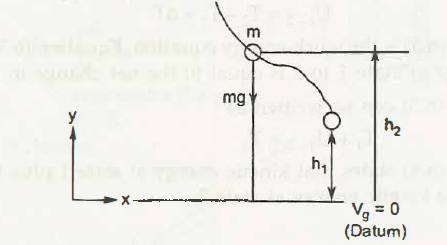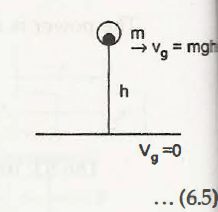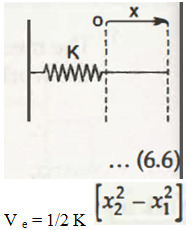Potential Energy is defined as the energy stored in an object because of its change in position and results in producing some work.

Mathematically, it is written as-

Work   = Force x Displacement

= mg x h

Or,

P.E. = mgh

Where,

m = Mass of the object

g = Gravity

mg = Weight of the object

h = Height or Change in Position

1. Gravitational Potential Energy

Gravitational Potential Energy can be defined as the force required for lifting an object from one position to another position as shown in the figure below.Let the initial position of the object is at h1 distance from datum

The final position it attains after applying the force is h2 from the datum

Mass of the object be m

Weight is mg

So, the gravitational potential energy, Vg can be calculated as-

Work done= dy

mgdy   = mg (h2 – h1)

=  mgh

Considering the figure below;∆Vg  =mg  = ∆h
Or,
Vg  =mgh

1. Elastic Potential Energy

Elastic Potential Energy can be defined as the energy stored in the elastic materials because of deformation. For example, stretching of spring where the magnitude of potential energy depends on the constant K (Spring Constant) and the distance it is stretched.

Let us consider x be the distance stretched, then considering the figure below, we get-Suppose the deformation will occur from x1 to x2, then

Elastic Potential Energy, Ve = ½ K (x22 – x12)

Links of Next Mechanical Engineering Topics:-### Customer Reviews

My Homework Help
Rated 5.0 out of 5 based on 510 customer reviews at